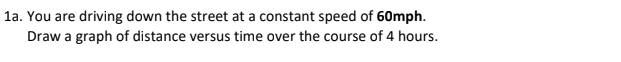# 1 You are driving down the street at a constant speed of 60mph. a. Draw a graph of distance versus time over the course of 4 hours. b. How would you use the graph to determine the rate (speed in mph) at which the car is travelling (assuming you did not already know the answer)? Hint: What is the slope for the graph in part A? What does the term slope mean?